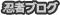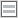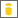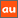# 0除算に関するアナウンスメントを公開しています

The purpose of this site is to publish announcements on the theory of division by zero

## Announcement 412: The 4th birthday of the division by zero $z/0 = 0$

Institute of Reproducing Kernels
Kawauchi-cho, 5-1648-16,
Kiryu 376-0041, Japan
February 2, 2018

The Institute of Reproducing Kernels is dealing with the theory of division by zero calculus and declares that the division by zero was discovered as $0/0 =1/0 = z/0 = 0$ in a natural sense on 2014.2.2. The result shows a new basic idea on the universe and space since Aristotels $($BC384 - BC322$)$ and Euclid $($BC 3 Century - $)$, and the division by zero is since Brahmagupta $($598 - 668?$)$. In particular, Brahmagupta defined as $0/0 = 0$ in Brhmasphuasiddhnta $($628$)$, however, our world history stated that his definition $0/0 = 0$ is wrong over 1300 years, but, we showed that his definition is suitable. For the details, see the references and the site: http://okmr.yamatoblog.net/ We wrote a global book manuscript  with 154 pages and stated in the preface and last section of the manuscript as follows:

Preface
The division by zero has a long and mysterious story over the world $($see, for example, H. G. Romig  and Google site with the division by zero$)$ with its physical viewpoints since the document of zero in India on AD 628. In particular, note that Brahmagupta $($598 -668 ?$)$ established the four arithmetic operations by introducing 0 and at the same time he defined as $0/0 = 0$ in Brhmasphuasiddhnta. Our world history, however, stated that his definition $0/0 = 0$ is wrong over 1300 years, but, we will see that his definition is right and suitable.
The division by zero $1/0 = 0/0 = z/0$ itself will be quite clear and trivial with several natural extensions of the fractions against the mysterously long history, as we can see from the concepts of the Moore-Penrose generalized inverses or the Tikhonov regularization method to the fundamental equation $az = b$, whose solution leads to the definition $z = b/a$.
However, the result $($definition$)$ will show that for the elementary mapping
$$W =\frac{1}{z} \tag{0.1}$$the image of $z = 0$ is $W = 0$ $($should be defined from the form$)$. This fact seems to be a curious one in connection with our well-established popular image for the point at infinity on the Riemann sphere (). As the representation of the point at infinity of the Riemann sphere by the zero $z = 0$, we will see some delicate relations between 0 and $\infty$ which show a strong discontinuity at the point of infinity on the Riemann sphere. We did not consider any value of the elementary function $W = 1/z$ at the origin $z = 0$, because we did not consider the division by zero $1/0$ in a good way. Many and many people consider its value by the limiting like $+\infty$ and $-\infty$ or the point at infinity as $\infty$. However, their basic idea comes from continuity with the common sense or based on the basic idea of Aristotle. – For the related Greece philosophy, see [23, 24, 25]. However, as the division by zero we will consider its value of the function $W = 1/z$ as zero at $z = 0$. We will see that this new definition is valid widely in mathematics and mathematical sciences, see $($[9, 10]$)$ for example. Therefore, the division by zero will give great impacts to calculus, Euclidean geometry, analytic geometry, differential equations, complex analysis in the undergraduate level and to our basic ideas for the space and universe.
We have to arrange globally our modern mathematics in our undergraduate level. Our common sense on the division by zero will be wrong, with our basic idea on the space and the universe since Aristotle and Euclid. We would like to show clearly these facts in this book. The content is in the undergraduate level.

Conclusion
Apparently, the common sense on the division by zero with a long and mysterious history is wrong and our basic idea on the space around the point at infinity is also wrong since Euclid. On the gradient or on derivatives we have a great missing since $tan(\pi/2) = 0$. Our mathematics is also wrong in elementary mathematics on the division by zero.
This book is an elementary mathematics on our division by zero as the first publication of books for the topics. The contents have wide connections to various fields beyond mathematics. The author expects the readers write some philosophy, papers and essays on the division by zero from this simple source book.
The division by zero theory may be developed and expanded greatly as in the author’s conjecture whose break theory was recently given surprisingly and deeply by Professor Qi’an Guan  since 30 years proposed in  $($the original is in $)$.
We have to arrange globally our modern mathematics with our division by zero in our undergraduate level.
We have to change our basic ideas for our space and world.
We have to change globally our textbooks and scientific books on the division by zero.

References
 L. V. Ahlfors, Complex Analysis, McGraw-Hill Book Company, 1966.
 L. P. Castro and S. Saitoh, Fractional functions and their representations, Complex Anal. Oper. Theory 7 $($2013$)$, no. 4, 1049-1063.
 Q. Guan, A proof of Saitoh’s conjecture for conjugate Hardy H2 kernels, arXiv:1712.04207.
 M. Kuroda, H. Michiwaki, S. Saitoh, and M. Yamane, New meanings of the division by zero and interpretations on 100/0 = 0 and on 0/0 = 0, Int. J. Appl. Math. 27 $($2014$)$, no 2, pp. 191-198, DOI: 10.12732/ijam.v27i2.9.
 T. Matsuura and S. Saitoh, Matrices and division by zero  $z/0=0$, Advances in Linear Algebra & Matrix Theory, 6$($2016$)$, 51-58 Published Online June 2016 in SciRes. http://www.scirp.org/journal/alamt http://dx.doi.org/10.4236/alamt.2016.62007.
 T. Matsuura and S. Saitoh, Division by zero calculus and singular integrals. $($Submitted for publication$)$
 T. Matsuura, H. Michiwaki and S. Saitoh, log0 = log∞ = 0 and applications. Differential and Difference Equations with Applications. Springer Proceedings in Mathematics & Statistics.
 H. Michiwaki, S. Saitoh and M.Yamada, Reality of the division by zero z/0 = 0. IJAPM International J. of Applied Physics and Math. 6$($2015$)$, 1–8. http://www.ijapm.org/show-63-504-1.html
 H. Michiwaki, H. Okumura and S. Saitoh, Division by Zero $z/0 = 0$ in Euclidean Spaces, International Journal of Mathematics and Computation, 28$($2017$)$; Issue 1,  1-16.
 H. Okumura, S. Saitoh and T. Matsuura, Relations of 0 and ∞, Journal
of Technology and Social Science $($JTSS$)$, 1$($2017$)$, 70-77.
 H. Okumura and S. Saitoh, The Descartes circles theorem and division by zero calculus. https://arxiv.org/abs/1711.04961 $($2017.11.14$)$.
 H. Okumura, Wasan geometry with the division by 0. https://arxiv.org/abs/1711.06947 International Journal of Geometry.
 H. Okumura and S. Saitoh, Applications of the division by zero calculus to Wasan geometry. $($Submitted for publication$)$.
 S. Pinelas and S. Saitoh, Division by zero calculus and differential equations. Differential and Difference Equations with Applications. Springer Proceedings in Mathematics & Statistics.
 H. G. Romig, Discussions: Early History of Division by Zero, American
Mathematical Monthly, Vol. 31, No. 8. $($Oct., 1924$)$, pp. 387-389.
 S. Saitoh, The Bergman norm and the Szegö norm, Trans. Amer. Math. Soc. 249 $($1979$)$, no. 2, 261–279.
 S. Saitoh, Theory of reproducing kernels and its applications. Pitman Research Notes in Mathematics Series, 189. Longman Scientific & Technical, Harlow; copublished in the United States with John Wiley & Sons, Inc., New York, 1988. x+157 pp. ISBN: 0-582-03564-3
 S. Saitoh, Generalized inversions of Hadamard and tensor products for matrices, Advances in Linear Algebra & Matrix Theory. 4 $($2014$)$, no. 2, 87–95. http://www.scirp.org/journal/ALAMT/
 S. Saitoh, A reproducing kernel theory with some general applications, Qian,T./Rodino,L.$($eds.$)$: Mathematical Analysis, Probability and Applications - Plenary Lectures: Isaac 2015, Macau, China, Springer Proceedings in Mathematics and Statistics, 177(2016), 151-182. $($Springer$)$.
 S. Saitoh, Mysterious Properties of the Point at Infinity、arXiv:1712.09467 [math.GM]$($2017.12.17$)$.
 S. Saitoh, Division by zero calculus $($154 pages: draft$)$: $($http://okmr.yamatoblog.net/ $)$
 S.-E. Takahasi, M. Tsukada and Y. Kobayashi, Classification of continuous fractional binary operations on the real and complex fields, Tokyo Journal of Mathematics, 38$($2015$)$, no. 2, 369-380.
 https://philosophy.kent.edu/OPA2/sites/default/files/012001.pdf
 http://publish.uwo.ca/ jbell/The 20Continuous.pdf
 http://www.mathpages.com/home/kmath526/kmath526.htm
 Announcement 179 $($2014.8.30$)$: Division by zero is clear as z/0=0 and it is fundamental in mathematics.
 Announcement 185 $($2014.10.22$)$: The importance of the division by zero $z/0 = 0$.
 Announcement 237 $($2015.6.18$)$: A reality of the division by zero $z/0 = 0$ by geometrical optics.
 Announcement 246 $($2015.9.17$)$: An interpretation of the division by zero $1/0 = 0$ by the gradients of lines.
 Announcement 247 $($2015.9.22$)$: The gradient of y-axis is zero and $\tan(\pi/2) = 0$ by the division by zero $1/0 = 0$.
 Announcement 250 $($2015.10.20$)$: What are numbers? - the Yamada field containing the division by zero z/0 = 0.
 Announcement 252 $($2015.11.1$)$: Circles and curvature - an interpretation by Mr. Hiroshi Michiwaki of the division by zero $r/0 = 0$.
 Announcement 281 $($2016.2.1$)$: The importance of the division by zero $z/0 = 0$.
 Announcement 282 $($2016.2.2$)$: The Division by Zero z/0 = 0 on the Second Birthday.
 Announcement 293 $($2016.3.27$)$: Parallel lines on the Euclidean plane from the viewpoint of division by zero $1/0=0$.
 Announcement 300 $($2016.05.22$)$: New challenges on the division by zero $z/0=0$.
 Announcement 326 $($2016.10.17$)$: The division by zero z/0=0 - its impact to human beings through education and research.
 Announcement 352$($2017.2.2$)$: On the third birthday of the division by zero $z/0=0$.
 Announcement 354$($2017.2.8)$:$  What are $n = 2,1,0$ regular polygons inscribed in a disc? – relations of 0 and infinity.
 Announcement 362$($2017.5.5$)$: Discovery of the division by zero as $0/0 = 1/0 = z/0 = 0$
 Announcement 380 $($2017.8.21$)$: What is the zero?
 Announcement 388$($2017.10.29$)$: Information and ideas on zero and division by zero $($a project$)$.
 Announcement 409 $($2018.1.29.$)$:  Various Publication Projects on the Division by Zero.
 Announcement 410 $($2018.1 30.$)$: What is mathematics? – beyond logic; for great challengers on the division by zero.

PR

### コメント

お名前 Black LimeGreen SeaGreen Teal FireBrick Tomato IndianRed BurlyWood SlateGray DarkSlateBlue LightPink DeepPink DarkOrange Gold DimGray Silverプロフィール

ＨＮ：